Chen, G.-F., and Liu, M.-H. (2012). "Adsorption of L-lysine from aqueous solution by spherical lignin beads: Kinetics and equilibrium studies," BioRes. 7(1), 298-314.

#### Abstract

The potential of spherical lignin beads as an adsorbent to take up L-lysine from aqueous solution was investigated. The kinetic data were estimated by the pseudo-first-order and pseudo-second-order models. The mechanism of adsorption was also studied using the Boyd model and Webber’s intraparticle diffusion model. The equilibrium data were modeled by the Langmuir, Freundlich, and Dubinin-Radushkevich (D-R) isotherms as well. Results suggested that the adsorption kinetics can be best described by the pseudo-first-order model. The equilibrium data could be well fitted using the Langmuir isotherm model with a maximum adsorption capacity of 67.11 mg·g-1. The adsorption characteristics of the spherical lignin adsorbent proved the feasibility of its use as an alternative adsorbent for the removal of L-lysine from aqueous solution.

Adsorption of L-lysine from aqueous solution by Spherical Lignin Beads: Kinetics and Equilibrium Studies

Guo-Fen Chen,a, b and Ming-Hua Liub,*

The potential of spherical lignin beads as an adsorbent to take up L-lysine from aqueous solution was investigated. The kinetic data were estimated by the pseudo-first-order and pseudo-second-order models. The mechanism of adsorption was also studied using the Boyd model and Webber’s intraparticle diffusion model. The equilibrium data were modeled by the Langmuir, Freundlich, and Dubinin-Radushkevich (D-R) isotherms as well. Results suggested that the adsorption kinetics can be best described by the pseudo-first-order model. The adsorption process initially was controlled by film diffusion, whereas the subsequent stage was controlled by intraparticle diffusion. The equilibrium data could be well fitted using the Langmuir isotherm model with a maximum adsorption capacity of 67.11 mg·g-1. The adsorption characteristics of the spherical lignin adsorbent proved the feasibility of its use as an alternative adsorbent for the removal of L-lysine from aqueous solution.

Keywords: Spherical lignin; Adsorption; L-lysine; Kinetic

Contact information: a: Department of Biological and Chemical Engineering, Fuqing Branch University of Fujian Normal University, Fuzhou, Fujian, 350300, China; b: College of Environment & Resources, Fuzhou University, Fuzhou, Fujian, 350108, China; *Corresponding author: mhliu2000@fzu.edu.cn

INTRODUCTION

As a representative essential amino acid for human and animal nutrition, L-lysine is economically used as a supplement in animal feed as well as in food, improving the quality of the feed and food by increasing the absorption of other amino acids. L-Lysine can also be used particularly as one of the key ingredients of infusion solutions. Additionally, it can be used as an effective medicine in clinical use. Griffith and his co-workers (Griffith et al. 1987) found that L-Lysine could be an effective agent in reducing the occurrence, severity, and healing time of the recurrent herpes simplex (HSV) infection. Chang and Gao (1995) reported that L-lysine could enhance the activity of diazepam against seizures induced by pentylenetetrazol (PTZ), and increase the affinity of benzodiazepine receptor binding in a manner additive to that caused by γ-aminobutyric acid (GABA). Liu and Zhang (2008) found that L-lysine enhances glucose-induced insulin secretion in insulin-producing cells (INS-1E) in a glucose concentration range of 1.1 to 25 mmol·L-1.

Chemical, enzymatic, and fermentation processes have been used to synthesize lysine (Shah 2002). For commercial production of L-lysine, fermentation processes have been more predominantly employed, and several hundred thousand tonnes of L-lysine (about 800,000 tonnes/year) are annually produced worldwide by bacterial fermentation (Savas 2007).

For many years, L-Lysine has been produced by fermentation, separation, puri-fication, and concentration processes. After fermentation, the resulting broth may be rendered cell free by filtration or centrifugation. The L-Lysine may then be recovered from the fermentation broth through ion exchange, and thereafter concentrated by evaporation and spray drying. L-lysine of resultant culture broth can be recovered by known conventional methods such as using ion exchange resins (Savas 2007). Most resins such as strong acidic cation exchange resins, weak acidic cation exchange resins, macroporous resins, and petroleum-based polymers, however, make this process expensive.

The present paper presents the results relating to the application of a spherical lignin adsorbent (denoted as SLA) for L-lysine adsorption from aqueous solution. Kinetic parameters were calculated to describe the adsorption mechanism of L-lys on SLA. The Langmuir, Freundlich, and Dubinin–Radushkevich (D-R) models were also used to describe the equilibrium isotherms.

EXPERIMENTAL

Materials

Black liquor containing Masson pine alkali lignin was kindly provided by the Fujian Nanping Paper Group Co. Ltd., Fujian, China. The transformer oil 10# was of industrial grade. L-lysine was a biological reagent (Chengdu Kolong Chemical Reagent Co., Chengdu, China). All other chemicals used, including epichlorohydrin, chloro-benzene, sodium lauryl benzenesulfate, sodium hydroxide, and hydrochloric acid, were chemically pure and from Shanghai Chemical Reagent Co., Shanghai, China.

Instruments

Laboratory devices used included a XL30 ESEM-TMP stereoscan (Philips-FEI Co, Holland), a SH10A moisture rapid determination instrument (Shanghai Hengping Instruments Co, China), a Spectrum 2000 FT-IR Spectrometer (Pekin-Elmer Co, USA), and a UV-2001 Recording Spectrophotometer (Shimadzu, Japan).

Spherical lignin adsorbent was prepared by a reverse-phase suspension polymer-ization method similar to that reported in the literature (Liu and Deng 2006). 120 mL of a mixture of transformer oil and chlorobenzene (1:1.5 of volume ratio) was used as the disperse phase. 50 g of black liquor (28.7% of lignin content) was then slowly added into the oil mixture in a 500 mL three-mouth flask. After stirring for 20 min at a speed of 250 r·min-1, the calculated amount of dispersant agent and epichlorohydrin as a cross-linking agent were slowly added into the reactor. The mixture was first stirred at 60 oC for 60 min before the reaction temperature was raised to 90 oC. After reacting for 60 min, the slurry was cooled to room temperature. The slurry was filtered to recover the disperse phase, washed with water then acetone, and then dried. Reddish-brown spherical lignin beads were obtained (denoted as SLA) and the dried sample was sieved to obtain a particle size range of 0.30 to 0.45 mm. The physicochemical properties of SLA are presented in Table 1 and SEM images of the SLA are shown in Fig. 1.

Table 1. Physicochemical Properties of the SLA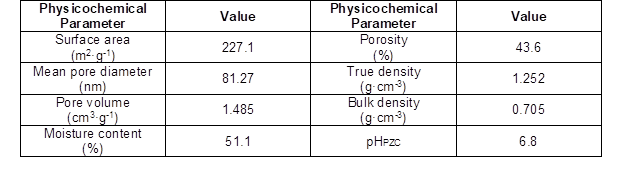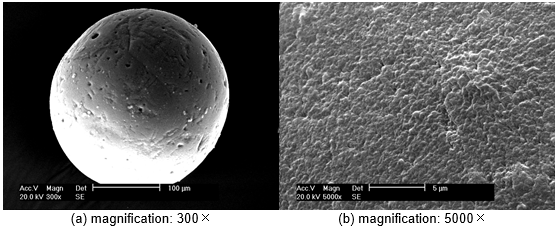Fig. 1. SEM images of the Spherical lignin bead

Equilibrium experiments were performed by adding predetermined amounts of SLA (0.5 g, 51.1% H2O) with 100 mL of aqueous L-lysine of a desired concentration (in the range of 100 to 700 mg·L-1), pH, and temperature in series to 250 mL glass-stoppered Erlenmeyer flasks. The mixtures were then shaken at 90 rpm at constant speed for predetermined time intervals. Equilibrium was reached after 150 min of shaking. The solute from each flask was then filtered out. The residual L-lysine concentrations in the liquid phase (Ce) were analyzed using the acidic ninhydrin method (Paul et al. 1995) with a model UV-2001 Recording Spectrophotometer (Shimadzu, Japan) at 570 nm. The equilibrium adsorption capacity of the SLA was calculated according to the following formula,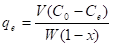(1)

where qe (mg·g-1) is the equilibrium adsorption capacity of the adsorbent; V (L) is the solution volume; W (g) is the adsorbent dosage; and x (%) is the moisture content of the adsorbent.

The batch study was set up according to previous studies (Liu et al. 2009). For kinetic studies, 0.50 g of SLA was added to 100 mL of L-lysine with a concentration of 300 mg·L-1 using a water-bath shaker at 25 oC. The agitation speed was kept constant at 90 rpm. At predetermined time intervals, the solutions were analyzed for the final concentration of L-lysine.

RESULTS AND DISCUSSION

Effect of Solution pH

The effect of solution pH on the adsorption capacity of L-lysine on SLA is presented in Fig. 2. Solution pH is an important controlling parameter in the adsorption process, and the role of solution pH was examined in solutions at different pH values covering a range of 3.0 to 11.0. Results show that L-lysine adsorption by SLA is highly dependent upon the solution pH. This suggests that L-lysine is adsorbed according to the ion-exchange mechanism. The equilibrium adsorption capacity was lowest at pH 3.0 (19.9 mg·g-1) and increased up to pH 5.0, reached a maximum (37.1 mg·g-1) over the initial pH 4.0 to 7.0. With an increase in solution pH ranging from 6.0 to 9.0, the adsorption capacity increased sharply from 33.0 mg·g-1 to 63.3 mg·g-1. Decreased adsorption was noticed as the pH was increased further from 10.0 to 12.0. The optimum pH for adsorption of L-lysine by SLA was observed at pH 9.0. Consequently, the solution pH was adjusted to 9.0 to conduct all the subsequent adsorption experiments.

L-lysine can be dissociated into four ionic states at different pH values: lys2+, lys+, lys±, and lys. Figure 2 (Kitadai et al. 2009) shows the changes in the dissociation states of lysine. The pHPZC of SLA was found to be 6.8 by a mass titration method (Noh and Schwarz 1989), indicating that the surface charge of the SLA is negative above 6.8, which facilitated the attraction of lys+ when pH ranged from 7.0 to 9.0. When the solution pH exceeded 9.0, the ion-exchange function of the negatively charged group with lys± became weak. Hence, the equilibrium adsorption capacity decreased in pH range from 9.0 to 10.0.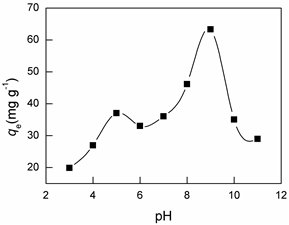Fig. 2. Effect of solution pH on adsorption of L-lysine on SLA. Sorption conditions: adsorbent usage, 0.5 g/100 mL; Co, 500 mg·L-1; adsorption time, 120 min; temperature, 25 oC (Liu et al. 2009)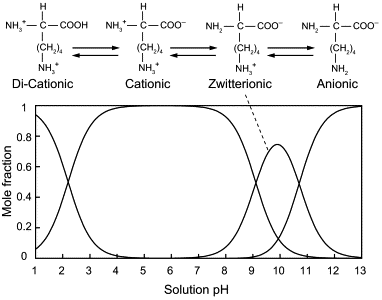Fig. 3. Changes as a function of pH in the mole fractions of different dissociation states of dissolved lysine, determined on the basis of published dissociation constants(pK a): α-carboxyl group (2.2), α-amino group (9.1), and side-chain-amino group (10.7) (Gambino et al. 2004; Kitadai et al. 2009 )

The FTIR spectrums of SLA before and after adsorption of L-lysine are shown in Fig. 4. For the SLA adsorbents, a strong band at 3423 cm-1 indicated the presence of hydroxyl groups, whereas bands in the 3000-2800 cm-1 region of the C–H stretch are attributed to the methoxyl groups (Albadarin et al. 2011). The characteristic bands located at 1464 and 1510 cm-1correspond to aromatic C=C in the samples, and the peak at 1267 cm-1 is attributed to C–OH (hydroxyl) bending of phenolic structure in lignin (Wu et al. 2008; Albadarin et al. 2011). It can be observed that after SLA reacted with the lignin functional groups, the sharp stretching vibration at 1593 cm-1 was broadened (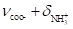) and the –NH2 bending bend (1558 cm-1) was absent or only very weak.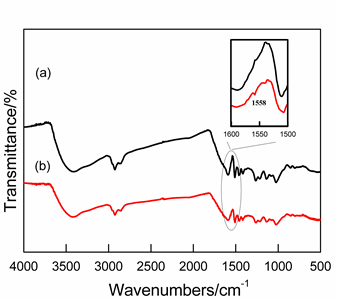Fig. 4. FTIR spectrum of SLA (a) before and (b) after L-lysine adsorption(pH=9.0)

The kinetic plots for the adsorption of L-lys onto SLA are presented in Fig. 5. The plots show the amount of L-lysine adsorbed at different time intervals with initial adsorbent concentrations of 300 mg·L-1 and 5 g·L-1.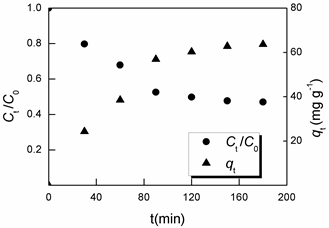Fig. 5. Kinetic plots for the adsorption of L-lysine onto SLA. Sorption conditions: adsorbent usage, 0.5 g/100 mL; pH, 9.0; temperature, 25oC

From the figure it can be observed that the adsorption capacity of L-lys gradually increased with an increase in adsorption time, followed by a slow process until the adsorption equilibrium was established after 150 min. At equilibrium time, the L-lysine adsorption capacity onto SLA was found to be 63.6 mg·g-1 and the Ct/C0 to be 47.0%.

The kinetic data were analyzed using Lagergren’s pseudo-first-order model (Eq. 2), and Ho’s pseudo-second-order model (Eq. 3) (Ho and McKay 1999).

The pseudo-first-order equation is represented in the following form,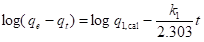(2)

The pseudo-second-order equation is expressed as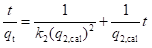(3)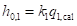(4)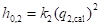(5)

where qe (mg·g-1) is the experimental amount of L-lysine adsorbed at equilibrium; qt (mg·g-1) is the amount of L-lysine adsorbed at time tk1 (min-1) is the equilibrium rate constant of first-order sorption; q1,cal (mg·g-1) is the adsorption capacity calculated by the pseudo-first-order model; h0,1 (mg·g-1·min-1) is the initial sorption rate calculated by the pseudo-first-order model (Eq. 4); k2 (g·mg-1·min-1) is the equilibrium rate constant of pseudo-second-order sorption; q2,cal (mg·g-1) is the adsorption capacity calculated by the pseudo-second-order kinetic model; and h0,2 (mg·g-1·min-1) is the initial sorption rate calculated by the pseudo-second-order kinetic model (Eq. 5).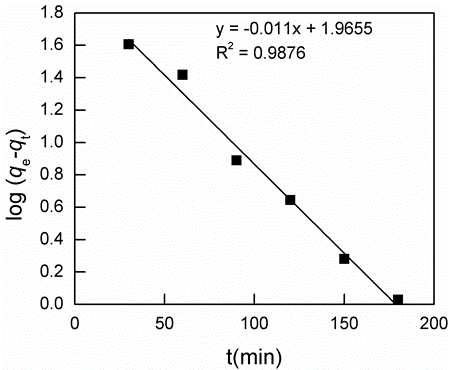Fig. 6. The pseudo-first-order kinetic model of the adsorption of L-lysine on SLA. Sorption conditions: adsorbent usage, 0.5 g/100 mL; pH, 9.0; temperature, 25oC

The fitting results are presented in Fig. 6 as a linear plot of log(qeqt) against t for the first-order model and in Fig. 7 as t·qt-1 versus t for the pseudo-second-order model. The calculated values of the rate parameters, adsorption capacity, and the regression coefficients are shown in Table 2. On the basis of correlation coefficients, it was observed that the experimental data was slightly better described by the first-orderkinetic model (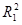=0.9876) than the second-order kinetic model (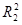=0.9566) indicating the applicability of the pseudo-first-order model.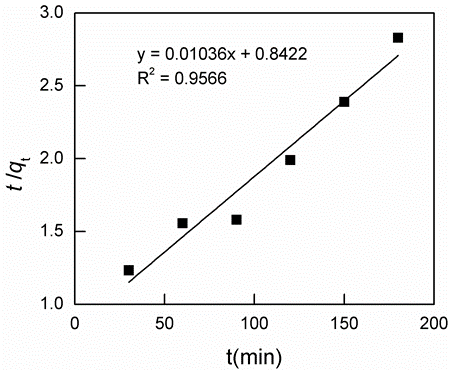Fig. 7. The pseudo-second-order kinetic model of the adsorption of L-lysine on SLA. Sorption conditions: adsorbent usage, 0.5 g/100 mL; pH, 9.0; temperature, 25 oC

Table 2. Kinetic Model Parameters for the Adsorption of L-lys on SLA at 25 oC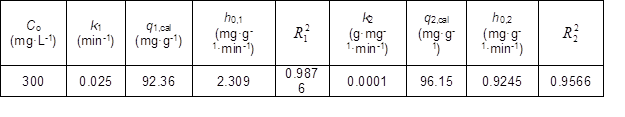To investigate the mechanism of adsorption of L-lysine on SLA, the kinetic data obtained for the adsorption were evaluated using Boyd’s kinetic model (Boyd et al. 1947) and Webber’s intraparticle model (Weber-Jr and Morris 1963).

Boyd’s model is expressed as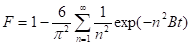(6)

The values of F can be determined by,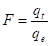(7)

where F is the fractional attainment of equilibrium at various times tqt (mg·g-1) and qe (mg·g-1) are the amounts adsorbed at time t and at equilibrium (150 min in the present study), respectively; and B (min-1) is Boyd’s constant.

Reichenberg (1953) modified Boyd’s kinetic expression by applying the Fourier transform, and its approximations are obtained by Eq. 8 and Eq. 9:

For F values>0.85，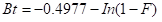(8)

And for 0<F values<0.85，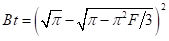(9)

For the valves of F calculated from Eq. 7, the corresponding valves of Bt could be obtained from Eq. 8 or Eq. 9. The linearity test of plots of Bt versus t (Fig. 8) was employed to predict the particle diffusion control mechanism. From Fig. 8 it was observed that the plots were linear but did not pass through the origin, signifying that film diffusion is the rate-limiting step.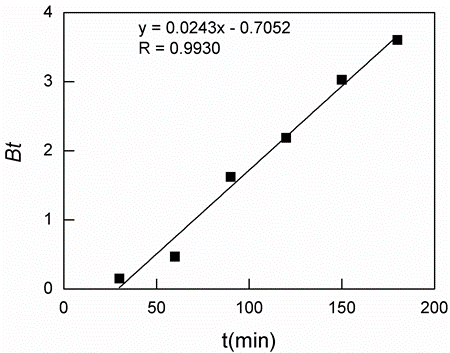Fig. 8. The Boyd plot for the adsorption of the L-lysine onto SLA. Sorption conditions: adsorbent usage, 0.5 g/100 mL; pH, 9.0; temperature, 25oC

The value of B was calculated from the slope of the straight line of the plot of Bt against shown in Fig. 8. B can be used to calculate the effective diffusion coefficient D(cm2·s-1) using the equation: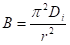(10)

where r is the radius of the adsorbent particle (that is assumed to be spherical) and calculated from sieve analysis. The Di was calculated as 3.1×10-8 cm2·s-1 (r: 270 μm).

On the other hand, Webber’s intraparticle diffusion equation can be written by the following equation,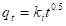(11)

where qt (mg·g-1) is the amount adsorbed at time t; and ki (mg·g-1·min-0.5) is the intraparticle diffusion rate constant. The ki values were calculated from the slopes of the straight line regions of the respective plots of qt against t 0.5.

Figure 9 shows the plot of qt versus t 0.5 on the basis of Webber’s intraparticle diffusion mechanism for the adsorption of L-lysine onto SLA. For the diffusion mechanism, if the plot was linear and passed through the origin, then the rate controlling step in the adsorption process would have been the internal diffusion, and vice versa.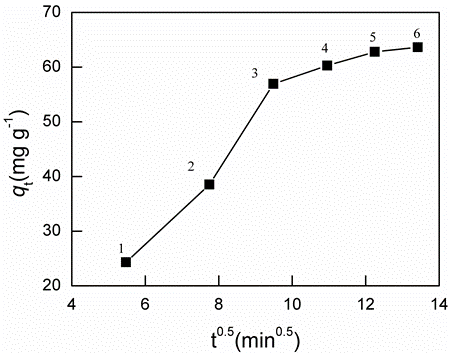Fig. 9. Intraparticle diffusion kinetic plots for the adsorption of the L-lys onto SLA. Sorption conditions: initial L-lys concentration, 300 mg·L-1; adsorbent usage, 0.5 g/100 mL; pH, 9.0; temperature, 25oC

The plot in Fig. 9 exhibited approximately four distinct sections. It was confirmed that the initial portion (initial two data points), was due to film diffusion, meaning that an external mass transfer took place from the solution to the adsorbent. It was followed by two linear segments, corresponding to the possible intraparticle diffusion, where the adsorbate molecules transferred to the interior of the adsorbent particles. The fourth stage was the plateau region and represented the equilibrium of the adsorption process. A similar trend was reported in the adsorption of pare-chlorophenol onto activated carbon (Koumanova et al. 2003). The rate controlling mechanism may vary during the course of the sorption process (Allen et al. 2005); thus, the adsorption process initially was controlled by film diffusion, whereas the subsequent stage was controlled by intraparticle diffusion in the present study. The rate parameter for the diffusion segment ki1 and ki2, theoretically, were related to the different diffusion rates attributed to the different pore sizes in terms of macropores, mesopores, and micropores (Allen et al. 1989). The rate parameter for the two diffusion segments, ki1 (for the 2rd and 3rd data points) and ki2 (for the data points from the 3rd to the 5th) can be calculated from the slopes of the straight line, respectively. The kvalues were calculated from the slopes of the straight line regions of the diffusion-plots of qt against t 0.5. The calculated values of the rate parameters are presented in Table 3.

Table 3. Parameters for DiffusionEquilibrium Isotherms

Equilibrium isotherm plots represent the equilibrium relationship of the adsorbate between the solute concentration in solution and in the adsorbed phase. In the present study, the Langmuir, Freundlich, and Dubinin-Radushkevich (Dubinin and Radushkevich 1947) equations were adopted to fit the equilibrium adsorption data. The Langmuir and Freundlich equations are the most widely used models. The Langmuir equation is obtained based upon an assumption of monolayer coverage over a homogenous adsorption surface, whereas the Freundlich isotherm is an exponential equation and is applicable to heterogeneous surface adsorption.

The Langmuir equation can be written in the following form: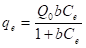(12)

The linear form of Eq. 12 is shown in Eq. 13 and used to determine constants Qo and b (Yuan et al. 2011):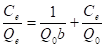(13)

The Freundlich equation has the following form (Yu et al. 2011):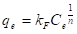(14)

The Langmuir constants, kF and 1/n, are evaluated through the linearization of Eq. 14,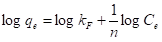(15)

where Ce (mg·L-1) is the equilibrium concentration; qe (mg·g-1) is the experimental amount of L-lysine adsorbed at equilibrium; Q0 (mg·g-1) is the theoretical monolayer capacity; b(L·mg-1) is the Langmuir constant; and kand n are the Freundlich constants, where n is related to the heterogeneity factor.

A concave-up type of isotherm was observed from the experimental data analyzed by the Freundlich and Langmuir equations and is presented in Fig. 10. The fitting results are also presented in Fig. 11 as a plot of Ce/qe versus t, in Fig. 12 as a plot of logqe versus Ce, and in Fig. 13 as a plot of logqe versus ε2. The calculated values of the Langmuir, Freundlich, and Dubinin–Radushkevich parameters and the regression coefficients are shown in Table 4. It can be seen that Langmuir isotherm fit the data slightly better than the Freundlich isotherm. This was also corroborated based on the coefficients of determination for the two isotherms, shown in Fig. 11 and Fig. 12, indicating monolayer adsorption taking place on a homogeneous adsorbent surface. The monolayer saturation capacity (Q0) of 67.11 mg·g-1 was obtained at temperature 25oC. This value was comparable to the adsorption capacities of various adsorbents for L-lysine (Table 5). The adsorption capacity of SLA was relatively high when compared to some other adsorbents reported in the literature. Consequently, it is feasible for the spherical lignin adsorbent to adsorb and purify L-lysine from aqueous solutions.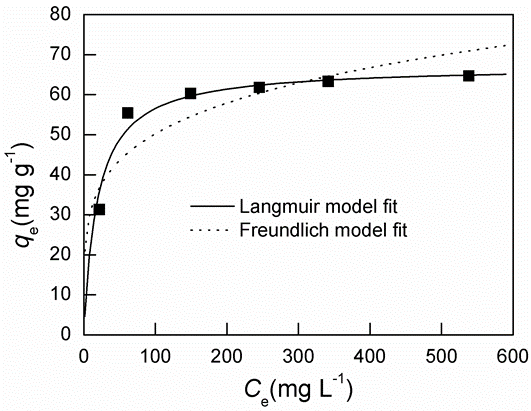Fig. 10. The equilibrium isotherm plots for the adsorption of L-lysine on SLA. Sorption conditions: adsorbent usage, 0.5 g/100 mL; pH, 9.0; temperature, 25oC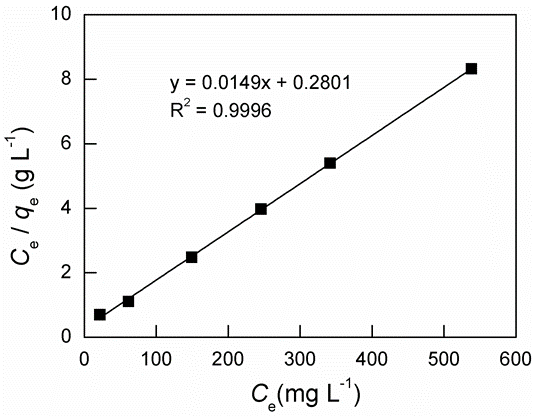Fig. 11. Linearized Langmuir model fit for the adsorption of L-lysine on SLA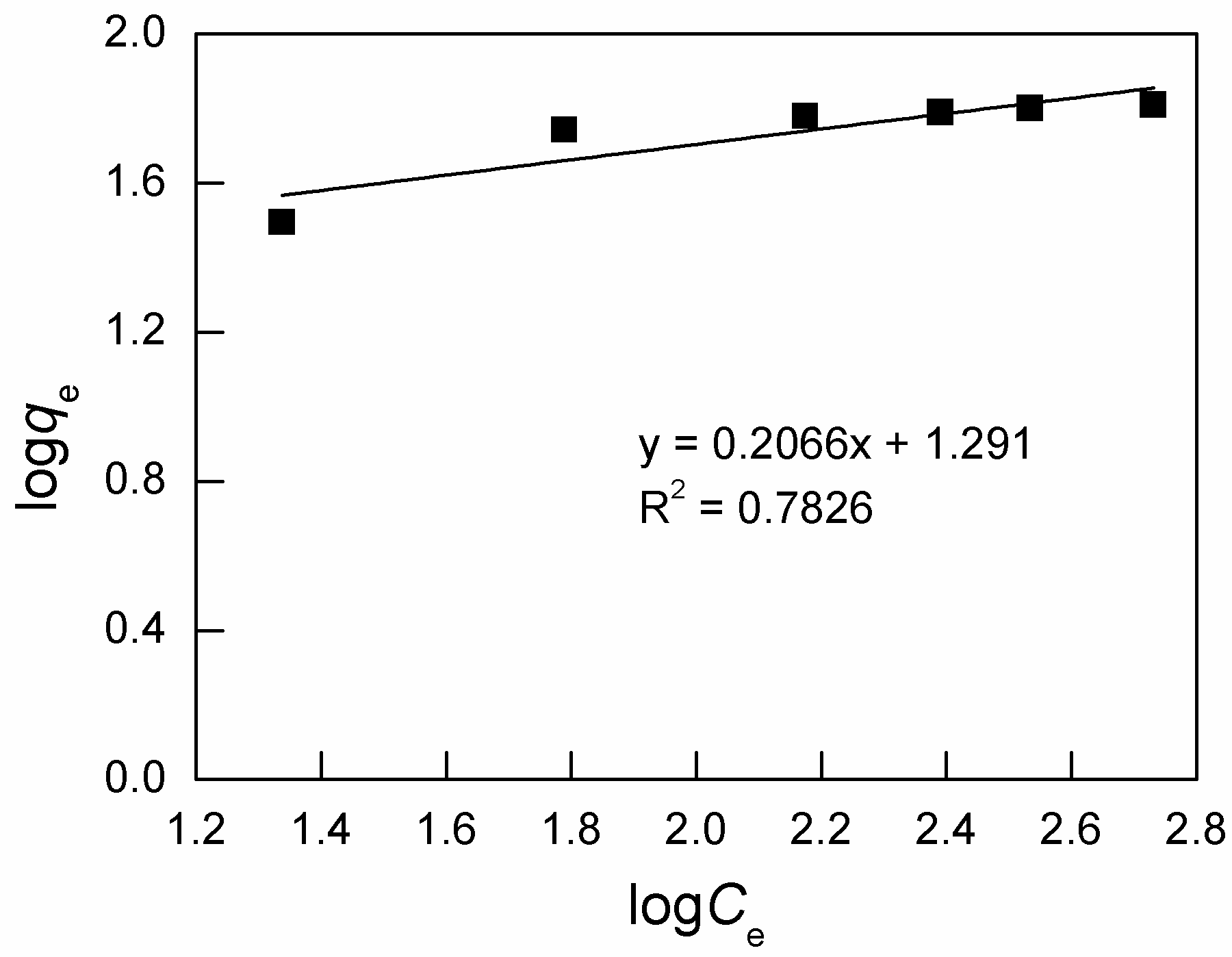Fig. 12. Linearized Freundlich model fit for the adsorption of L-lysine on SLA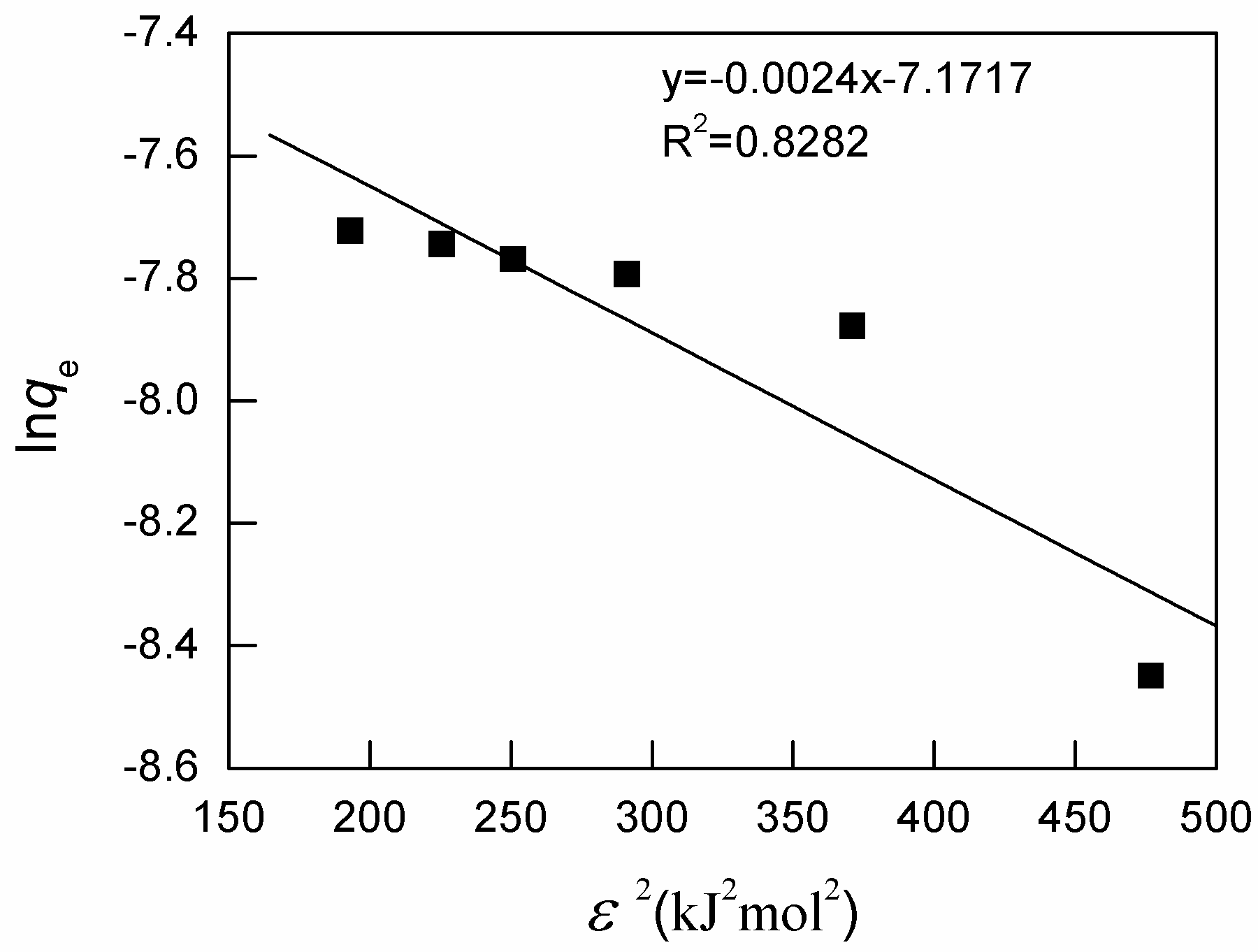Fig. 13. Linearized D-R model fit for the adsorption of L-lysine on SLA

Table 4. Isotherm Parameters for the Adsorption of the L-lysine onto SLA at 25oC (C0=300 mg·L-1)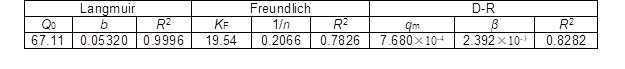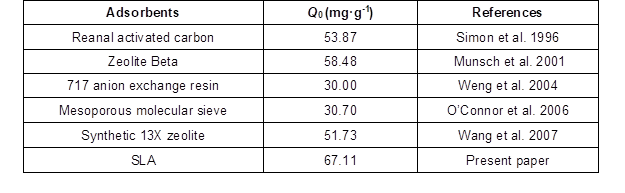The essential feature of the Langmuir equation can be expressed in terms of a dimensionless separation factor of equilibrium parameter, RL, which is defined by (Weber and Chakravorti 1974),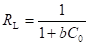(16)

where b is the Langmuir constant introduced in Eq. 11 and Eq. 12 and C0 is the initial concentration of the adsorbate in solution.

The RL value indicates the favorability characteristic for the adsorption system, i.e., favorable (0<RL<1), unfavorable (RL>1). The RL value obtained was 0.059 indicating a favorable adsorption process.

On the other hand, the D-R equation is represented as (Dubinin and Radushkevich 1947),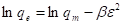(17)

where qm (mol·g-1) is the theoretical saturation capacity; ε (KJ·mol-1) is the Polanyi potential, which is numerically equal to RT In[1+（1/Ce）], where R (J·mol-1·K-1) is the gas constant, T (K) is the absolute temperature, and Ce (mol·L-1) is the equilibrium L-lysine concentration in solution; and β (mol2·KJ-2) is the similarity constant.

The calculated values of the Dubinin-Radushkevich constants and regression coefficients are given in Table 4. The plots in Fig. 12 and Fig. 13 indicate that the D-R equation agrees well with the Freundlich model over moderate concentration ranges, but not for the initial stage (indicated from the value of lnqe in Fig. 13). Furthermore, the mean free energy of adsorption (E) was calculated by the following equation (Dubey and Gupta 2005; Hasany and Chaudhary 1996):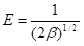(17)

The mean adsorption energy E was calculated to be 14.43 kJ·mol-1 (E > 8 kJ/mol), signifying that a chemical ion-exchange mechanism was potentially involved in the adsorption process for the initial stage (Onyango et al. 2004; Srivastava and Hasan 2011).

CONCLUSIONS

1. The mechanism of L-lysine adsorption on SLA in an aqueous medium was investi-gated on the basis of kinetic and equilibrium studies. The equilibrium time was found to be 150 min for the adsorption of L-lysine on SLA. The adsorption kinetics can be better described by the pseudo-first-order model. It was found that the adsorption process for the initial period was controlled by film diffusion, whereas the subsequent stage was controlled by intraparticle diffusion.
2. The equilibrium data were analyzed by the Langmuir, Freundlich, and D-R isotherm models. The Langmuir adsorption isotherm provided the best correlation fitting to the experimental data, suggesting monolayer adsorption on a homogenous surface. The mean adsorption energy was calculated and signified that a chemical ion-exchange mechanism was potentially involved through the adsorption process.
3. The adsorption behavior of SLA and its physicochemical properties proved the feasibility of using the alkali lignin material as an adsorbent for the adsorption of L-lysine from an aqueous solution.

ACKNOWLEDGMENTS

The research was financially supported by National Science Foundation of China (21077024) and Foundation of Fuzhou University (0460-022326).

REFERENCES CITED

Albadarin, A. B., Al-Muhtaseb, A. H., Al-laqtah, N. A., Walker, G. M., Allen, S. J., and Ahmad, M. N. M. (2011). “Biosorption of toxic chromium from aqueous phase by lignin: mechanism, effect of other metal ions and salts,” Chemical Engineering Journal 169, 20-30

Allen, S. J., McKay, G., and Khader, K. Y. H. (1989). “Intraparticle diffusion of a basic dye during adsorption onto sphagnum peat,” Environ. Pollut. 56, 39-50.

Allen, S. J., Koumanova, B., Kircheva, Z., and Nenkova, S. (2005). “Adsorption of 2-nitrophenol by technical hydrolysis lignin: Kinetics, mass transfer, and equilibrium studies,” Ind. Eng. Chem. Res. 44, 2281-2287.

Boyd, G. E., Adamson, A. W., and Myers-Jr, L. S. (1947). “The exchange adsorption of ions from aqueous solutions by organic zeolites, II: Kinetics,” J. Am. Chem. Soc. 69, 2836-2848.

Carrillo-M. G., Davila-J., M. M., Elizalde-G., M. P., and Palaez-C., A. A. (2001). “Removal of metal ions from aqueous solution by adsorption on the natural adsorbent CACMM2,” Journal of Chromatography A 938(1-2), 237-242.

Chang, Y. F., and Gao, X. M. (1995). “L-lysine is a barbital-like anticonvulsant and modulator of the benzodiazepine receptor,” Neurochem Res. 20(8), 931.

Dubey, S. S., and Gupta, R. K. (2005). “Removal behavior of Babool bark (Acacia nilotica) for submicro concentrations of Hg2+ from aqueous solutions: A radiotracer study,” Sep. Purif. Technol. 41(1), 21-28.

Dubinin, M. M., and Radushkevich, L. V. (1947). “Equation of the Characteristic Curve of activated charcoal, Proceedings of the Academy of Sciences,” Proceedings of the Academy of Sciences of the USSR, Chemistry Section 55, 331-333.

Gambino, G. L., Lombardo, G. M., Grassi, A., and Marletta, G. (2004). “Molecular modeling of interactions between l-lysine and a hydroxylated quartz surface,” J. Phys. Chem. B 108, 2600-2607.

Griffith, R. S., Walsh, D. E., Myrmel, K. H., Thompson, R. W., and Behforooz, A. (1987). “Success of L-lysine therapy in frequently recurrent herpes simplex infection,” Dermatologica175(4), 183-190.

Hasany, S. M., and Chaudhary, M. H. (1996). “Sorption potential of Haro River sand for the removal of antimony from acidic aqueous solution,” Applied Radiation and Isotopes 47(4), 467-471.

Ho, Y. S., and McKay, G. (1999). “A kinetic study of dye sorption by biosorbent waste product pith,” Res. Conserv. Recycl. 25(3-4), 171-193.

Kitadai, N., Yokoyama, T., and Nakashima, S. (2009). “ATR-IR spectroscopic study of L-lysine adsorption on amorphous silica,” Journal of Colloid and Interface Science 329, 31-37.

Koch, H. F., and Roundhill, D. M. (2001). “Removal of mercury(II) nitrate and other heavy metal ions from aqueous solution by a thiomethylated lignin material,” Sep. Sci. Technol. 36(1),137-43.

Koumanova, B., Peeva, P., and Allen, S. J. (2003). “Variaton of intraparticle diffusion parameter during adsorption of p-chlorophenol onto activated carbon made from apricot stones,” J. Chem. Technol. Biotechnol. 78, 582-587.

Liu, M.-H., Chen, G.-F., Yao, M.-B., and Yang, J. (2009). “The adsorption performance of L-lysine on a spherical lignin adsorbent,” Transactions of China Pulp and Paper 24(1), 72-75.

Liu, M.-H., and Deng, Y. (2006). “Adsorption of L-arginine from aqueous solutions on spherical sulfonic lignin adsorbent,” American Laboratory 38(2), 18-22.

Liu, M.-H., and Huang, J.-H. (2006). “Removal and recovery of cationic dyes from aqueous solutions using spherical sulfonic lignin adsorbent,” Journal of Applied Polymer Science101(4), 2284-2291.

Liu, M.-H., Huang, J.-H., and Deng, Y. (2007). “Adsorption behaviors of l-arginine from aqueous solutions on a spherical cellulose adsorbent containing the sulfonic group,” Bioresource Technology 98(5), 1144-1148.

Liu, M.-H., Hong, S.-N., Huang, J.-H., and Zhan, H.-Y. (2005). “Adsorption/desorption behavior between a novel amphoteric granular lignin adsorbent and reactive red K-3B in aqueous solutions,” Journal of Environmental Sciences 17(2), 212-214.

Liu, Zh. P., and Zhang, Y. L. (2008). “The acute effect of L-lysine on clonal insulin-producing cell Lines,” Tianjin Med. J. 36(9), 663-665.

Munsch, S., Hartmann, M., and Ernst, S. (2001). “Adsorption and separation of amino acids from aqueous solutions on zeolites,” Chem. Commun. 19, 1978-1979.

Noh, J. S., and Schwarz, J. A. (1989). “Estimation of the point of zero charge of simple oxides by mass titration,” J. Colloid Interface Sci. 130, 157-164.

O’Connor, A. J., Hokura, A., Kisler, J. M., Shimazu, S., Stevens, G. W., and Komatsu,Y. (2006). “Amino acid adsorption onto mesoporous silica molecular sieves,” Separation and Purification Technology 48, 197-20.

Onyango, M. S., Kojima, Y., Aoyi, O., Bernardo, E. C., and Matsuda, H. (2004). “Adsorption equilibrium modeling and solution chemistry dependence of fluoride removal from water by trivalent-cation-exchanged zeolite F-9,” J. Colloid Interface Sci. 279(2), 341-350.

Paul, L. H., Ben, K. S., and Jocelyn, R. E. (1995). “Amino acids interfere with the ninhydrin assay for asparagine,” Food Chemistry 53(4), 467-469.

Reichenberg, D. (1953). “Properties of ion-exchange resins in relation to their structure, III. Kinetics of exchange,” J. Am. Chem. Soc. 75(3), 589-597.

Savas, A. (2007). “L-Lysine Fermentation,” Recent Patents on Biotechnology. 1(1), 11-24.

Shah, A. H., and Hameed, A. (2002). “Fermentative production of L-lysine: fungal fermentation and mutagenesis-II: A review,” Pak. J. Pharm. Sci. 15(2), 29-35.

Simon, G., Hanak, L., Grévillot, G., Szanya, T., and Marton, G. (1996). “Amino acid separation by preparative temperature-swing chromatography with flow reversal,” Journal of Chromatography A 732, 1-15.

Srivastava, P., and Hasan, S. H. (2011). “Biomass of Mucor heimalis for the biosorption of cadmium from aqueous solutions: Equlibrium and kinetic studies,” BioResources 6(4), 3656-3675.

Sun, Y., Zhang, J.-P., Yang, G., and Li Z.-H. (2005). “Removal of chlorophenol in waste solution by activated carbon produced from reed pulp lignin by precarbonization,” Mordern Chemical Industry 26, 226-229.

Wang, Y.-B., Jiang, B., Li J.-J., and Xiao, W. (2007). “Experimental studies of the lysine treatment in wastewater with 13X zeolite,” Acta Petrologica Et Mineralogica 26(1), 90-94.

Weber-Jr, W. J., and Morris, J. C. (1963). “Kinetics of adsorption on carbon from solution,” J. Sanitary Eng. Div. Proceed. Am. Soc. Civil Eng. 89, 31-59.

Weber, T. W., and Chakravorti, R. K. (1974). “Pore and solid diffusion models for fixed-bed adsorbers,” AIChE Journal 20(2), 228-238.

Weng, L. J., Gan, L. H., Wang, S. B., Han, Y. Y., and Li, X. L. (2004). “Adsorption Characteristics of L-lysine on 717 Anion Exchange Resin,” Ion Exchange and Adsorption 20(2), 119-124.

Wu, Y., Zhang, S., Guo, X. Y., and Huang, H. L. (2008). “Adsorption of chromium(III) on lignin,” Bioresource Technology 99 (16), 7709-7715.

Yuan, G., Dai, H., Ye, C., Zhang, Y., and Wang, Z. (2011). “Adsorption of Ca(II) from aqueous solution onto cellulosic fibers and its impact on the papermaking process,” BioResources6(3), 2790-2804.

Yu, X., Zhang, G., Xie, C., Yu, Y., Cheng, T., and Zhou, Q. (2011). “Equilibrium, kinetic, and thermodynamic studies of hazardous dye neutral red biosorption by spent corncob substrate,”BioResources 6(2), 936-949.

Article submitted: September 5, 2011; Peer review completed: October 23, 2011; Revised version received and accepted: November 11, 2011; Published: November 14, 2011.